### Home > CCA > Chapter 8 > Lesson 8.1.5 > Problem8-49

8-49.
1. Factor each polynomial. Homework Help ✎

1. x2 − 64

2. y2 − 6y + 9

3. 4x2 + 4x + 1

4. 5x2 − 45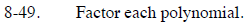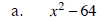This is a difference of two squares.

(x + 8)(x − 8)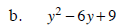This is a perfect square trinomial.

(y − 3)2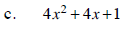Look at the hint in part (b).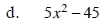Start by factoring out the greatest common factor. Then look at the hint in part (a).